# Waves emit particles

### Wave properties of electrons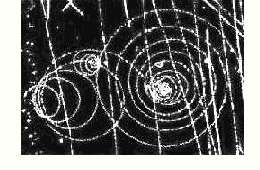After Robert Millikan determined the charge of the electron in 1911, its rest mass could soon also be measured. Numerous experiments with electron beams such as deflection in electric and magnetic fields spoke in favor of the corpuscular (to lat. corpusculum: "Particle") nature of electrons. In the picture on the right we see an image of electron traces in a bubble chamber. Even the presence of such traces indicates the particle nature of the electrons. As with all charged particles, the external magnetic field created spiral paths.

In the previous chapters we learned that light has both wave and particle properties, depending on the experimental conditions.

Then why shouldn't Reasons of symmetry Moving matter particles, i.e. objects with mass such as electrons, and vice versa also show wave properties?

Louis de Broglie 1923 was the first to propose a wave theory of particles in his doctoral thesis. The experiments with light gave us two relationships between the particle sizes E, p and the wave sizes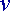,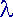: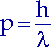De Broglie hypothesized that this relationship applies not only to photons, but also to electrons and other material particles:

De Broglie's hypothesis:

1. A wave with a wavelength belongs to every moving particle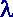= h / p.

2. Between the frequencythe wave and the energy E. of the particle the relation holds.

For an experimental proof of his "Matter waves" suggested de Broglie: If a particle beam crosses a very small opening, then diffraction phenomena should occur. The width of this opening is critical. Because the diffraction and at the same time also the interference at the slit are only noticeable during wave processes when the diameter of the slit is slightly larger than the wavelengthis. (For the diffraction angleof the first secondary maximum applies: sin=/ dd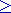.)

In order to obtain the diffraction and interference of electrons, a slit or a grid has to be found with an opening width that is insignificantly larger than the wavelength of the electrons in flight.

For non-relativistic velocities we can determine the wavelength of the moving particles as a function of their rest mass m0 bring. We set the momentum p = mv. We get the velocity v from the well-known formula E.kin = mv / 2.(7.1)

An electron that is accelerated by a voltage receives the kinetic energy E.kin = mv / 2 = eU. So we can write the wavelength of the electron as a function of voltage:(7.2)

At a voltage in the order of magnitude of 10V, electrons have a wavelength in the nanometer range, which is also the distance between atoms in solids. We remember: X-rays with wavelengths in the nanometer range are scattered by crystalline solids on their space lattice.

Crystals can thus also serve as diffraction grids for electrons!

Three years after de Broglie's hypothesis resulted Clinton Davisson and Lester Germer the first evidence of electron diffraction from:

Experiment by Davisson and Germer

Electrons emerge from the hot cathode and are accelerated by a voltage to the anode. After hitting a nickel crystal, they are scattered. The distance d between the atoms in the nickel crystal is 0.215 nm. The intensity of the scattered electrons is measured with a detector. The location of the detector is varied until the intensity reaches a maximum.
At an accelerating voltage of 54 V, the 1st order interference maximum appears at the angle= 50°.

We can now calculate the wavelength of the electrons in two ways:

1. Using an equation from optics, the following applies to the position of the maxima during diffraction on the grating:(7.3)

For the 1st order maximum (first secondary maximum) n = 1. The experiment gives us the following wavelength: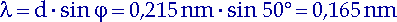(7.4)

 Wavelength from the experiment Atomic distance angle wavelength 0.215 nm 50° 0.165 nm

2. With de Broglie's relation (7.2) we can calculate the wavelength solely from the knowledge of its energy or the accelerating voltage: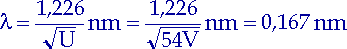(7.5)

 De Broglie wavelength tension wavelength 54 V 0.167 nm

With other voltages too, Davisson and Germer achieved a good agreement between the measured wavelength and the predicted wavelength of de Broglie.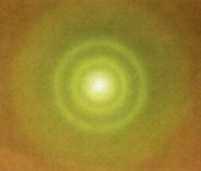In a similar experiment with a Electron diffraction tube we can visualize the interference of electrons on a fluorescent screen. Electrons emerge from the glowing cathode and are caused by the voltage UB. accelerated towards the anode. They reach the fluorescent screen through a hole in the anode and create a light spot there. If we put a thin graphite foil in between, then several concentric rings will form around the original light spot. Let us increase the voltage UB. so the rings get smaller. Because according to de Broglie we reduce the wavelength of electrons and with the formula (7.3) from optics the scattering angle also becomes smaller.

In further experiments with crystalline substances, protons and neutrons wave properties were also demonstrated.

The diffraction experiments on crystals confirmed de Broglie's hypothesis: Moved Micro-objects with rest mass, such as electrons, protons and neutrons, have wave properties.

Now we want to check the objects from our everyday life for such properties.

Is interference also observed with macro objects?

Does a flexion figure arise when throwing a ball through a window? So we have to find out whether the window width is on the order of the wavelength. We assume 1kg for the mass of the ball and 10 m / s for its speed.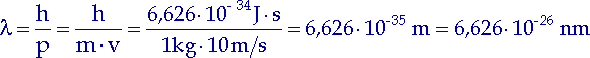(7.6)

Even for the subatomic area this value is very tiny, let alone for our ball. We see that objects from our detectable environment are too heavy to be able to observe wave properties. However, they can be calculated for any object.

Every form of matter has wave properties. But they do not play a role in our tangible world.

Shortly after the discovery of the wave properties of electrons, the assumption arose that electrons instead of light were used to enlarge and depict the smallest objects. This is because the distance that can be resolved with a microscope is directly proportional to the wavelength. In contrast to light quanta, electrons do not have a specific wavelength range and, according to de Broglie, can be brought to much smaller wavelengths by high voltages. The picture is setting schematically electron microscope Electrons accelerated by high voltage are bundled with the help of a magnetic coil and hit a thin object. When they pass through the object, they are scattered somewhat and therefore focused by a second coil. The third magnetic coil takes on the role of the eyepiece in the light microscope. It projects the electron beam onto a fluorescent screen or photo plate.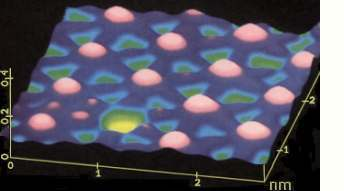A creates particularly vivid and high-contrast images Scanning tunnel microscope. The surface of the specimen is scanned with a fine electron beam. The picture shows us an image of iodine atoms (shown in pink) that are adsorbed on a platinum surface. The purple lines indicate bonds between the atoms. The yellow gap was created by removing an iodine atom.